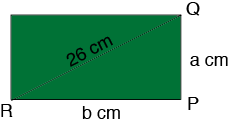SEARCH HOMEMath Central Quandaries & QueriesQuestion from niniha, a student: the perimeter of a rectangle is 68cm.if the diagonal is 26, find its dimensionsHi,

Step one. Draw a diagram.Since the perimeter is 68 cm what is $a + b?$

Triangle $PQR$ is a right triangle. What does Pythagoras theorem tell you?

Solve for $a$ and $b.$

PennyMath Central is supported by the University of Regina and the Imperial Oil Foundation.